RUSSIAN JOURNAL OF EARTH SCIENCES, VOL. 15, ES2002, doi:10.2205/2015ES000549, 2015

# Ionospheric response to annular and partial solar eclipse of 29 April 2014 in Antarctica and Australian Regions

Roshni Atulkar, Parvaiz A. Khan, Hussain Jeevakhan, P. K. Purohit

National Institute of Technical Teachers' Training and Research, Bhopal, MP, India

.

### Abstract

An annular and partial solar eclipse was observed on 29 April 2014 over Australian and Antarctic regions. In this study we have analyzed the ionospheric response of this solar eclipse event. We have done a comprehensive study to find out the changes that occurred in various ionospheric parameters during the solar eclipse event over Australia and Antarctic region. We selected four Australian stations Brisbane (27.5° S, 152.9° E), Canberra (35.3° S, 149.1° E), Hobart (42.9° S, 147.3° E) and Perth (31.955° S, 115.859° E) as well as one Antarctic station Mawson (70.6455° S, 131.2573° E). We have studied the changes in the $E$ and $F$ ionospheric layers using the ground based observations at these stations. From our analysis we found that there occurred a decrease in the critical frequencies of sporadic $E$ $(f_oE_s)$ and $F$ $(f_oF2)$ layers during the time eclipse was in progress at all the four Australian stations while as at Antarctic the value of $f_oF2$ recorded an enhancement. At the same time an increase in the corresponding heights of these layers ($h'E_s$, $h'F2$) was also observed.

### 1. Introduction

Solar eclipse is one of the important solar terrestrial events which have a direct impact on Earth's ionosphere. A solar eclipse provides us with a rare opportunity to study the ionospheric effects associated with an accurately estimated variation of solar radiation during the eclipse period. The ultraviolet radiations from the sun are blocked during solar eclipse, the ions and electrons start to recombine, therefore, the number of electrons and ions decrease. Hence compared to a normal day the loss rate of plasma on an eclipse day dominates over production rate. Thus, during solar eclipse lower electron density in the ionosphere is expected.

A comparative study of the different ionospheric parameters has revealed very interesting features. The temporal variation of $h'F$, $f_oF2$ and $f_oF1$ on the eclipse day has also been carried out by many workers earlier. It has been found that $h'F$ shows an oscillatory behavior during the course of the eclipse. In comparison with temporal variation of $f_oF2$ on the control days it drops by about 15% on the eclipse day. On the other hand $f_oF1$ decreases by as much as 50% on the eclipse day with no time lag between the time of maximum obscuration and the time of maximum decline of $f_oF1$ [Rama Rao et al., 1997].

Reduction of 20% was noticed both in $f_{\rm min}$ and in the $F1$ layer critical frequency during total solar eclipse of 11 August 1999. The signal strengths of the oblique incidence paths also point to eclipse associated decreases in ionization in the $D$ and lower $E$-region [Chandra et al., 2007]. Sporadic $E$ is a thin layer with dense patches of ionization around $E$ region altitudes, sporadic $E$ is generally observed between height of 95 km and 120 km. The $E_s$ structure and the related winds and wind shears for the formation of $E_s$ at magnetic equator (Thumba, dip 2° N) were studied in detail by [Sridharan et al., 1989]. [Chen et al., 2010] described the observations of sporadic $E$ ($E_s$) layer behavior over east China during the total solar eclipse. Sequential sporadic $E$ layers at low latitude in the Indian sector were presented by [Jayachandran et al., 1999] by comparing (Waltair, dip 20°) with (Thumba, dip 2° N) and (SHAR dip 10°). They provided the experimental evidence for the wind shear theory for the formation of descending night time sporadic $E$ layers by using three ionosonde data. They concluded that the night time descending sporadic $E$ layers are produced by the combined effect of the equator ward propagating gravity wave and the increased pole ward neutral wind which brings the ionization downward through the field-line [Veenadhari et al., 2002]. The $E_s$ consistently occurs around 100 km and the ordinary critical frequency of $E_s$ layer ($f_oE_s$) is unpredictable. The value of $f_oE_s$ is many times higher than its mean value [Chavdarov et al., 1968]. For the shadowing $E_s$-layer that occurred on 22 and 23, July, 2009 the blanketing frequency $f_bE_s$ was approximately equal to the critical frequency $f_oE_s$ [Piggott and Rawer, 1961; Reddy and Rao, 1968]. The sudden increase of foEs in the solar eclipse period was also observed over Haringhata [Datta, 1972, 1973]. It is worth noting that when the solar radiation decreases during totality the maximum electron concentration of $E_s$ did not fall but rose. The critical frequency of the $E$-layer was not measurable beyond 1600 h IST on eclipse day due to the strong blanketing sporadic-$E$, but 20% decrease in the critical frequency of the $F1$-layer and the minimum virtual height of the $F$-layer on eclipse day, decrease in the height of maximum ionization ($h_pF2$) during the eclipse, indicating a reduction in the thickness of the $F$-layer [Chandra et al., 2007]. The ionosphere $E$ and $F1$ layers are affected more than the $F2$ region during a solar eclipse [Rishbeth and Garriott, 1969]. The electron concentration value observed by [Nayak and Tiwari, 2012] they found no appreciable changes in $F2$ layer whereas 30% reduction observed in the $f_oF1$ during the maximum phase of eclipse.

### 2. Observation and Data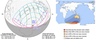Figure 1

An annular and partial solar eclipse occurred on 29 April 2014. A solar eclipse occurs when the moon passes between Earth and the sun, thereby totally or partly obscuring the image of the sun for a viewer on earth. An annular solar eclipse occurs when the moon's apparent diameter is smaller than the sun's, blocking most of the solar radiation and causing the sun to look like an annular (ring). An annular eclipse appears as a partial eclipse over a region of the Earth thousands of kilometers wide. The path of the eclipse and the area that had been covered by the eclipse shadow is shown in Figure 1. The solar eclipse was first observed at the Antarctica at exactly 05:00 UT and moved across Australian regions, Canberra (06:08 UT), Brisbane (06:31 UT), Perth (06:42 UT), and Hobart (07:00 UT).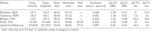Table 1

To investigate the ionospheric response of this solar eclipse we have selected four ionosonde stations in the Australian region and one station in the Antarctic region over which the eclipse event was observed. The location, eclipse timings and maximum obstructions of the selected ionosonde stations is provided in Table 1. The enhancement/decrement of the ionospheric parameters at each station are also provided in this table.

The ionosonde data used in the study was taken from National Geophysical Data Center (NGDC) database under URL: http://spidr.ngdc.noaa.gov/spidr/. NGDC maintains a huge database of ionosonde data recorded over more than 250 ionosonde stations. The data of different ionospheric parameters is provided in different time resolutions. However, we have taken the values of $f_oE_s$, $f_oF2$, $h'E_s$ and $h'F2$ with 15 minute resolution. To quantify the eclipse effect we have calculated the changes in these parameters denoted as $\Delta f_oE_s$, $\Delta f_oF2$, $\Delta h'E_s$ and $\Delta h'F2$. The changes on the eclipse day were calculated from the median of control days i.e. by subtracting the control day median from the eclipse day median. In order to isolate and associate the ionospheric effects with the eclipse we have checked for the solar and geomagnetic disturbances. To characterize the geomagnetic and solar activity levels we have used few indices ($Dst$, $Ap$, $Kp$) taken from Space Physics Data Facility (SPDF) at http://omniweb.gsfc.nasa.gov.

### 3. Results and Discussion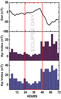Figure 2

In order to associate the ionospheric effects with the eclipse event and to confirm that these effects were not associated with solar or geomagnetic disturbances we have checked for the variation of geomagnetic indices during the eclipse and control days and is presented in the Figure 2. From Figure 2 it can be seen that a magnetic disturbance of moderate intensity with minimum $Dst$ of $- 67$ nT was observed on 30 April 2014. The value of $Kp$ index and $Ap$ index also recorded an increase with peak values 8.6 and 62 respectively indicating an increased geomagnetic activity. However, the eclipse day was a completely quiet day as indicated by the lowest values of $Kp$ and $Ap$ indices. Therefore, the observed ionospheric effects were not due to geomagnetic disturbance.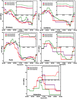Figure 3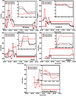Figure 4

In order to assess the effect of annular solar eclipse on ionospheric parameters, an estimate must be made of the variation of these parameters in the absence and presence of eclipse. Observations on the eclipse day i.e. 29 April 2014 were compared with those of the one day each before and after the eclipse day chosen as the control days. One of the important parameters of $F$ layer that is vulnerable to eclipse event is the critical frequency of $F2$ layer ($f_oF2$). Consequently, we have studied the temporal variation of $f_oF2$ on the eclipse day and compared it with the control days. Figure 3 shows the time profile of $f_oF2$ on the eclipse day and two control days at all the five selected stations. In the inset we have incorporated the variation of $f_oF2$ during the eclipse time at each station. From the Figure 3 we find a decrease occurred in the $f_oF2$ during the eclipse time compared to two control days at Brisbane, Canberra, Perth and Hobart while an increase in $f_oF2$ was observed at Antarctic during the eclipse time compared to control days. The amount of decrease and increase at each station in provided in Table 1. Since the amount of solar radiation incident on the ionosphere decreases, the ionospheric ionization also decreases resulting decrease in electron density and hence $f_oF2$ decreases. At the same we also investigated the change in the height of the $F$ layer. Figure 4 shows the temporal variation of $h'F2$ during the control days and the eclipse day at all the five selected stations. From the figure we find that an increase in $h'F2$ occurred during eclipse time compared to control days at Brisbane, Perth and Hobart while a decrease in $h'F2$ was recorded at Canberra and Antarctic. The amount of decrease and increase at each station is provided in Table 1.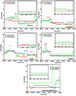Figure 5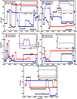Figure 6

We then investigated the eclipse induced changes in the $E$ layer ionosphere by considering $f_oE_s$ and $h'E_s$ parameters. Figure 5 shows the time profile of $f_oE_s$ on the eclipse and control days at all the selected stations. From this figure we find the value of $f_oE_s$ decreased during the eclipse time compared to the control days at all the selected stations. The amount of decrease at each station is provided in Table 1. The amount of decrease in $f_oE_s$ is more than the amount of decrease in $f_oF2$. Also we investigated the changes that occurred in the height of $E_s$ i.e. $h'E_s$ at each station on the eclipse and compared it with the control days. The temporal changes that occurred in the $h'E_s$ on 28, 29 and 30 April 2014 are shown in Figure 6. A significant increase in height was found during the eclipse time at all the station except at Canberra where a decrease in height was recorded during the eclipse time.

### 4. Conclusion

Analysis of the ionospheric parameters at 5 selected ionosonde stations located at Australian and Antarctica during the annular solar eclipse on 29 April 2014 showed the occurrence of depletion in the $f_oF2$, $f_oE_s$ and an enhancement in the corresponding heights of these layers $h'F2$ and $h'Es$. The conclusion of the study was, as the layer heights of $E$ and $F$ layer are increased, there was a corresponding decrease in critical frequencies of these layers. The behavior of $f_oF2$ and $h'F2$ was different at Australian and Antarctic stations.

#### Acknowledgments

The author (RA) would like to thank National Institute of Technical Teachers' Training and Research, Bhopal (MP), India for MHRD fellowship, National Geophysical Data Center's (NGDC), Space Physics Interactive Data Resource (SPIDR) website at http://spidr.ngdc.noaa.gov/spidr/, National Aeronautics and space Administration (NASA) eclipse service (http://eclipse.gsfc. nasa.gov/eclipse.html), and Omni Data centre website http:// omniweb.gsfc.nasa.gov, for providing data.

### References

Chandra, H., S. Sharma, P. D. Lele, G. Rajaram, A. Hanchinal (2007), Ionospheric measurements during the total solar eclipse of 11 August 1999, Earth Planets and Space, 59, p. 59–64, doi:10.1186/BF03352023.

Chavdarov, S. S., S. P. Chernysheva, A. M. Svechnikov (1968), Influence of radio wave absorption in the ionosphere on the variation of sporadic $E$ layer parameters, Geomagn. Aeron., 8, p. 294–298.

Chen, G., Z. Zhao, C. Zhou, G. Yang, Y. Zhang (2010), Solar eclipse effects of 22 July 2009 on sporadic-$E$, Journal of Annales Geophysicae, 28, p. 353–357, doi:10.5194/angeo-28-353-2010.

Datta, R. N. (1972), Solar eclipse effect on sporadic-$E$ ionization, Journal of Geophysical Research, 77, no. 1, p. 260–262, doi:10.1029/JA077i001p00260.

Datta, R. N. (1973), Solar eclipse effect on sporadic-$E$ ionization, 2, Journal of Geophysical Research, 78, no. 1, p. 320–322, doi:10.1029/JA078i001p00320.

Jayachandran, P. T., P. Sriram, P. V. S. Rama Rao, et al. (1999), Sequential sporadic-E layers at low latitudes in the Indian sector, Journal of Annales Geophysicae, 17, p. 519–525, doi:10.1007/s00585-999-0519-1.

Nayak, C. K., D. Tiwari, K. Emperumal, A. Bhattacharyya (2012), The equatorial ionospheric response over Tirunelveli to the 15 January 2010 annular solar eclipse: observations, Ann. Geophys., 30, p. 1371–1377, doi:10.5194/angeo-30-1371-2012.

Piggott, W. R., K. Rawer Eds. (1961), URSI Handbook of Ionogram Interpretation and Reduction, 324 pp., Elsevier, Amsterdam.

Rama Rao, P. V. S., D. S. V. V. D. Prasad, P. Sri Ram, et al. (1997), Ionospheric changes observed over Waltair (Dip 20° N) during the total solar eclipse of 24th October 1995, TAO, 8, no. 2, p. 203–212.

Reddy, C. A., M. M. Rao (1968), On the physical significance of the $E_s$ parameters $f_bE_s$, $fE_s$ and $f_oE_s$, Journal of Geophysical Research, 73, no. 1, p. 215–224.

Rishbeth, H., O. K. Garriott (1969), Introduction of Ionospheric Physics, 331 pp., Academic Press, New York.

Sridharan, R., R. Raghavaro, R. Suhasini, et al. (1989), Winds, wind shears and plasma densities during the initial phase of a magnetic storm from equatorial latitudes, Journal of Atmospheric and Terrestrial Physics, 51, p. 169–177, doi:10.1016/0021-9169(89)90099-8.

Veenadhari, B., R. S. Dabas, V. K. Vohra, D. R. Lakshmi, N. K. Sethi, S. C. Garg (2002), Study on Sporadic $E$ occurrences observed at New Delhi with Modern Digital Ionosonde, Proc. GA, 2, p. 0783.

Received 2 May 2015; accepted 4 May 2015; published 15 May 2015.Citation: Atulkar Roshni, Parvaiz A. Khan, Hussain Jeevakhan, P. K. Purohit (2015), Ionospheric response to annular and partial solar eclipse of 29 April 2014 in Antarctica and Australian Regions, Russ. J. Earth Sci., 15, ES2002, doi:10.2205/2015ES000549.

Generated from LaTeX source by ELXpaper, v.1.3 software package.